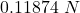Question

You are exploring a small moon of a distant planet. Measurements indicate that this moon is a sphere of uniform density rho = 5000 kg/m3. You dig a very deep hole into the moon that reaches within r0 = 1000 m of the center of the moon. With your spacesuit, you have a mass of m = 85 kg. Find the force of gravity on you when you stand at the bottom of the hole.

1.MichaelMet

The force of gravity will be “0.11874 N

Explanation:

The given values are:

Density of planet,

Q = 5000 Kg/m³

r₀ = 1000 m

Now,

The mass of inside sphere will be:

⇒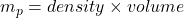⇒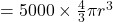On putting the values, we get

⇒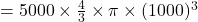⇒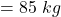hence,

The force of gravity will be:

=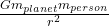=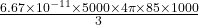=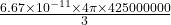=